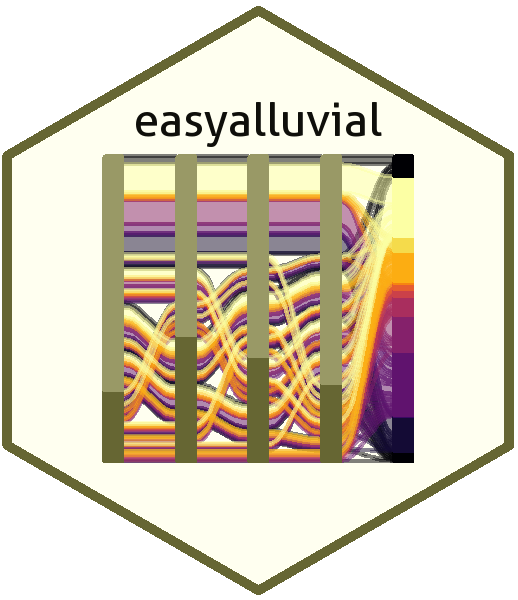# easyalluvialAlluvial plots are similar to sankey diagrams and visualise categorical data over multiple dimensions as flows. Rosval et. al. 2010 Their graphical grammar however is a bit more complex then that of a regular x/y plots. The `ggalluvial` package made a great job of translating that grammar into `ggplot2` syntax and gives you many option to tweak the appearance of an alluvial plot, however there still remains a multi-layered complexity that makes it difficult to use ‘ggalluvial’ for explorative data analysis. ‘easyalluvial’ provides a simple interface to this package that allows you to produce a decent alluvial plot from any dataframe in either long or wide format from a single line of code while also handling continuous data. It is meant to allow a quick visualisation of entire dataframes with a focus on different colouring options that can make alluvial plots a great tool for data exploration.

## Features

• plot alluvial graph with a single line of code of a given dataframe
• support for wide and long data format (wiki, wide vs. long/narrow data)
• automatically transforms numerical to categorical data
• helper functions for variable selection
• convenient parameters for coloring and ordering

## Installation

### CRAN

``install.packages('easyalluvial')``

### Development Version

``devtools::install_github("erblast/easyalluvial")``

## Tutorials

In order to learn about all the features an how they can be useful check out the following tutorials:

## Examples

### Alluvial from data in wide format

#### Prepare sample data

``````
suppressPackageStartupMessages( require(tidyverse) )
suppressPackageStartupMessages( require(easyalluvial) )

data = as_tibble(mtcars)
categoricals = c('cyl', 'vs', 'am', 'gear', 'carb')
numericals = c('mpg', 'cyl', 'disp', 'hp', 'drat', 'wt', 'qsec')

data = data %>%
mutate_at( vars(categoricals), as.factor )``````

#### Plot

Continuous Variables will be automatically binned as follows.

• High, High (HH)
• Medium, High (MH)
• Medium (M)
• Medium, Low (ML)
• Low, Low (LL)
``````
alluvial_wide( data = data
, max_variables = 5
, fill_by = 'first_variable' )``````### Alluvial from data in long format

#### Sample Data

``````
tailnum carrier origin dest qu mean_arr_delay
N0EGMQ LGA BNA MQ MQ LGA BNA Q1 on_time
N0EGMQ LGA BNA MQ MQ LGA BNA Q2 on_time
N0EGMQ LGA BNA MQ MQ LGA BNA Q3 on_time
N0EGMQ LGA BNA MQ MQ LGA BNA Q4 on_time
N11150 EWR MCI EV EV EWR MCI Q1 late
N11150 EWR MCI EV EV EWR MCI Q2 late

#### Plot

``````
alluvial_long( quarterly_flights
, key = qu
, value = mean_arr_delay
, id = tailnum
, fill = carrier )``````